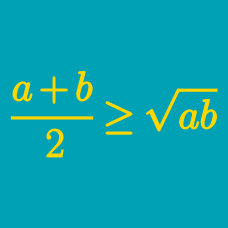Algebra

# Applying the Arithmetic Mean Geometric Mean Inequality

What is the smallest possible value of the expression $\frac{x^2+361}{x}$ for positive real $x$?

What is the minimum value of $y=\frac{x^2}{x-9}$ when $x>9$?

How many ordered pairs of real numbers satisfy

$36 ^ { x^2 + y} + 36 ^{ y^2 + x } = \frac{2} {\sqrt{6} } ?$

Let $y_1, y_2, y_3, \ldots, y_{8}$ be a permutation of the numbers $1, 2, 3, \ldots, 8$. What is the minimum value of $\displaystyle \sum_{i=1}^8 (y_i + i )^2$?

If $a$ and $b$ are positive numbers such that $a\ne 1, b\ne 1$ and $a+b \neq 1,$ what is the order relation of the following $3$ expressions $A, B$ and $C?$ \begin{aligned} A &= \frac{1}{\log_a 2}+\frac{1}{\log_b 2}, \\ B &= 2\left(\frac{1}{\log_{a+b} 2}-1\right), \\ C &= 2\left(1+\frac{1}{\log_{ab} 2}-\frac{1}{\log_{a+b} 2}\right) \end{aligned}

×